# The vertices of a triangle are (0,0) - Quantitative Aptitude - Geometry

## CAT 2020 - Slot 3 - Quantitative Aptitude - Geometry - Question 19 - The vertices of a triangle are (0,0)

Q. 19: The vertices of a triangle are (0,0), (4,0) and (3,9). The area of the circle passing through these three points is

##### 1.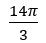2.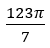3.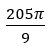4.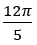C.

A circle is circumscribed on the points (0,0) (4,0) and (3,9)

We are asked to find the area of this circle

We know that the area of a triangle with sides a, b and c, inscribed ina circle of radius R is given by

Area =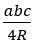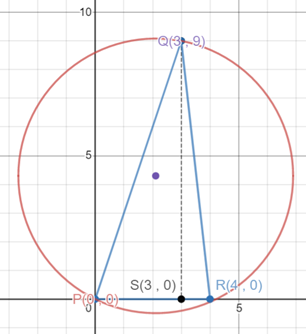With the help of teh co-ordinates of the triangle it is easy to find the height of the triangle, which is QS = 9 units.
Hence the area of the triangle = A = 1/2 × base × height = 1/2 × PR × QS = 1/2 × 4 × 9 = 18 sq. units
a = PR = 4 units
b = QR = √(QS2 + SR2) = √(92 + 12) = √82
c = PQ = √(PS2 + PQ2) = √(32 + 92) = √90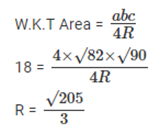Now that we know the radius of the circle the area of the circle = π × R2 = π ×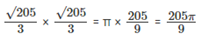### Past Year Question Paper & SolutionsCounselling Session
By IIM Mentor

#### Free Material Area